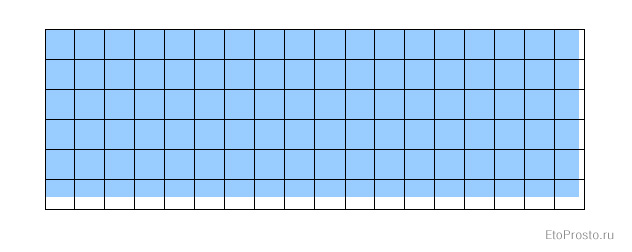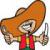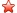RU
\\\The number of tiles per square meter. Area of a single tile

# The number of tiles per square meter. Area of a single tile

Usually tile is sold in square meters.  If you would like to order floor tile, all you need to know is the footage of the room: for instance if the area of your kitchen is 10 sq. meters, you will be ordering 10 m of tile + some extra for the borders and trim (usually around 10 percent).

Nevertheless, every once in a while, it is necessary to come up with the exact number of tiles used for each square meter.  If you want to visually imagine the picture and would like to estimate the number of tiles it would take per square meter (whether it would be 4, 10, or 20 tiles) it is fairly easy to figure out.  If, however, you need to calculate the exact number of tiles you need to purchase, in order to cover a square meter in your building, it is a tad bit more difficult. (That’s right, these two numbers can differ from each other a bit)

Let’s start with the easy part: the number of tiles in a square meter (completely abstract, any meter)

Calculating the area of a single tile

Initially, you need to calculate the area of a single tile in square meters. In order to do this, take the length of the tile (in meters) and multiply it by the width (in meters).  It is best to multiply in meters, instead of centimeters, so you do not get confused while converting square centimeters into square meters.

Let’s take, for example, a tile of 20x20 cm size. Since there are 100 centimeters in one meter, 20 cm is 0.2 meters (20/100=0.2).  We are going to multiply the length by the width 0.2m x 0.2m= 0,04 sq. m. Therefore, the tile of 20x20 cm size has an area of 0.04 sq. m.

If the tile size is 30 cm by 60 cm, the calculations indicate 0.3m x 0.6m=0.18 sq. m. For the tile that is 12,5cm x 120cm the area is going to be 0.125m x 1.2m=0.15sq. m.

 If you do not yet know what tile you would like to purchase and what size it has to have, please read The size of the floor tile article, in it you will find some of the most popular sizes. Be careful since, at times, the actual size of the tile does not match advertised parameters. For instance, the tile that is said to be 10x10 cm is actually 9.85 x 9.85 cm in size, and the tile that is supposedly 60 x 60 cm is 59.8 x 59.8 cm in actuality, etc.  For more details please read What is the caliber of tiles and why it is important to pay attention to it.

Counting the number of tiles

Now that you have the area of a single tile, all you need to do is divide one sq. meter by this area and you will get the number of tiles. So for the 20x20 cm tile the calculations are going to look like this: 1 sq. m / 0.04 sq. m = 25. Therefore, in one square meter there will be exactly 25 tiles that are 20x20 cm in size.

For the tile that is 30x60 cm the results are going to be the following:  1/0.18 = 5,555(5). So you will have about five and a half tiles.

 The number of tiles per square meter for some of the most popular tile sizes  (with some rounding up) 10x10 cm tile – 100 tiles per square meter 15x15 cm tile – 44.44 tiles per square meter 20x20 cm tile – 25 tiles per square meter 20x50 cm tile – 10 tiles per square meter 25x50 cm tile – 8 tiles per square meter 25x55 cm tile – 7.27 tiles per square meter 30x30 cm tile – 11.11 tiles per square meter 30x56 cm tile – 5.95 tiles in square meter 30x60 cm tile – 5.55 tiles in square meter 33x33 cm tile – 9 tiles in square meter 40x40 cm tile – 6.25 tiles per square meter 45x45 cm tile – 4.93 tiles per square meter 50x50 cm tile – 4 tiles per square meter 60x60 cm tile – 2.77 tiles per square meter

It is important to know that the estimated amount is an approximate one. There might be a different number of tiles per square meter in reality. Let’s take a look at why this might be the case.

Let’s say that our room is one meter by one meter in size (that same square meter area) and the tile we have is 30x60 cm. According to the preliminary calculations we need five and a half tiles, right? In reality we might need 7 tiles.Why does this happen?  It happens because our calculations take into account the entire area of the material, as if we would have laid it out in tiny pieces or poured it onto the surface. If you wanted to, of course, you could use the left over pieces from tiles 4, 5, and 6 to cover the area of the seventh tile. According to the estimations, there should be enough to do so. However, the length of left over pieces is less than the one we need (they are about 20 cm), therefore, the final result will look less than perfect.The bad news is that, most likely, we are going to need 8 tiles since we want the final product to look nice. Small pieces look rather awkward, thus we are going to even out the center.Well, you might decide that the problem lies in the fact that we picked the wrong size tile (30x60 cm) for such a small area (one sq. meter).  No one does this, and it is better to pick small tile. All right, let’s take the tile that is 10x10 cm. Nothing bad can happen with that, right? It takes exactly 100 tiles per one square meter (1/0.001=100).Well, not really.

The problem is that the room is not necessarily square with one meter by one meter in size. In actuality it could be a narrow hallway that is 2 meters by 50cm. Better yet, it could be a passageway with the exact parameters of 57 cm by 1.76 m. The tile we have is instantly going to give us left overs. In the end, this area is going to take 108 tiles. 18 tiles horizontally and 6 vertically.Of course, as the footage increases, the percentage of extra tiles usually goes down. In any case, prior to calculating the number of tiles, first identify the reason behind such calculations: is it simply to orient yourself or do you need the exact number for the order. If you need the exact number of tiles for the order – you cannot divide the area of the room by the area of the tile.  You must either make a drawing and orient yourself that way, or divide the length of the room by the length of the tile, the width of the room by the width of the tile and decide on ways to work with left overs.

Cost of the bathroom tile. Inexpensive tile

Cost of the bathroom tile. High quality imported tile

 Tweet

Просмотров: 216770Автор:
Mexica +9

Cредняя оценка: 5 | Всего голосов: 1 |Комментарии:
 Гость ( IP: 197.*.*.253 ) | 19.12.2019 14:06 This breakdown was very helpful. Thank you so much! Ответить | |
 Гость ( IP: 141.*.*.171 ) | 22.07.2018 14:26 thanks alot u really dont know how important this info is to me... Ответить | |
 Гость ( IP: 193.*.*.249 ) | 24.04.2018 09:56 Thanks for this information. It has really help me with estimating the number of tiles needed and costing. Ответить | |
 Гость ( IP: 109.*.*.197 ) | 12.10.2017 15:00 thank you very much Ответить | |
Написать комментарий:
Вы не авторизованы. При отправке сообщения, в качестве автора будет указан "Гость". Вход | Регистрация
Я не робот

Rubrics